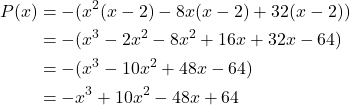Question

Find the polynomial of minimum degree, with real coefficients, zeros at x=4+4i and x=2, and y-intercept at 64

1.minhkhoi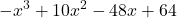Step-by-step explanation:

We want to find the minimum-degree polynomial with real coefficients and zeros at: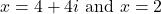As well as a y-intercept of 64.

By the Complex Root Theorem, if a + bi is a root, then abi is also a root.

So, a third root will be 4 – 4i.

The factored form of a polynomial is given by: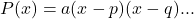Where a is the leading coefficient and p and q are the zeros. More factors can be added if necessary.

Substitute: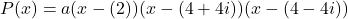Since we want the minimum degree, we won’t need to add any exponents.

Expand the second and third factors: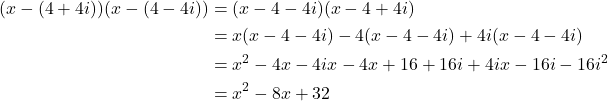Hence: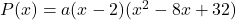Lastly, we need to determine a. Since the y-intercept is y = 64, this means that when x = 0, y = 64. Thus: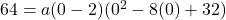Solve for a: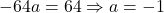Our factored polynomial is: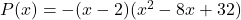Finally, expand: